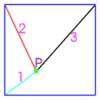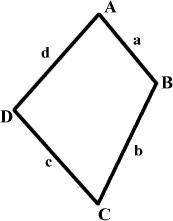#### You may also like### Just Touching

Three semi-circles have a common diameter, each touches the other two and two lie inside the biggest one. What is the radius of the circle that touches all three semi-circles?### Square World

P is a point inside a square ABCD such that PA= 1, PB = 2 and PC = 3. How big is angle APB ?### 30-60-90 Polypuzzle

Re-arrange the pieces of the puzzle to form a rectangle and then to form an equilateral triangle. Calculate the angles and lengths.

# Biggest Bendy

##### Age 16 to 18 Challenge Level:

Four rods are hinged at their ends to form a convex quadrilateral with fixed side lengths. Show that the quadrilateral has a maximum area when it is cyclic. Sue Liu from Madras College, St Andrews used two methods to solve this.

 ${\bf METHOD}$ ${\bf 1}$ First she applied Brahmagupta's formula for the area of a quadrilateral: $\delta = \sqrt{(s - a)(s - b)(s - c)(s - d) - abcd \cos^2 \beta}$ where $s = \frac{1}{2}(a + b + c + d)$ and $\beta = \frac{1}{2}(A + C)$ or $\frac{1}{2}(B + D)$. Hence the area is clearly the greatest when $abcd \cos^2 \beta$ is least. Since $\cos^2 \beta$ is always positive, this value is least when $\beta$ is $90$ degrees as $\cos 90^{\circ} = 0$. Hence $\frac{1}{2}(A + C) = 90$ and so $A + C = 180$ showing that the opposite angles in the quadrilateral add up to $180^{\circ}$ and so the area of a quadrilateral with fixed lengths of sides is greatest when it is cyclic. This method gives a proof of the required result but you have to assume Brahmagupta's formula and Sue's second method uses only the formula for the area of a triangle. ${\bf METHOD}$ ${\bf 2}$ The area of the quadrilateral $ABCD$ can be expressed as the sum of the areas of triangle $ABD$ and $BCD$. Let the area of $ABCD$ be $\Delta$. Then $\Delta = \frac{1}{2} ad\sin A + \frac{1}{2} bc \sin C$ Thus $$\frac {d\Delta}{dA} = \frac{1}{2} ad\cos A + \frac{1}{2} bc \cos C \frac {dC}{dA}.\quad (1)$$ From the Cosine Rule, $$a^2 + d^2 - 2ad\cos A = b^2 + c^2 - 2bc\cos C,$$ Hence, (differentiating both sides with respect to $A$), $$2ad\sin A = 2bc\sin C \frac {dC}{dA}.\quad (2)$$ From (1) and (2), \eqalign{ \frac{d\Delta}{dA} = \frac{1}{2} ad\cos A + \frac{1}{2} bc \cos C{ad\sin A \over bc\sin C}\\ = \frac {ad\cos A \sin C + \sin A \cos C}{2\sin C}\\ = \left(\frac{ad}{2}\right) \frac{\sin (A + C)}{\sin C} }. Hence, for the maximum area, $\sin (A + C)=0$ and $A+C=\pi$ which makes the quadrilateral cyclic. We can show that this gives the maximum and not the minimum value of $\Delta$ by finding the second derivative.